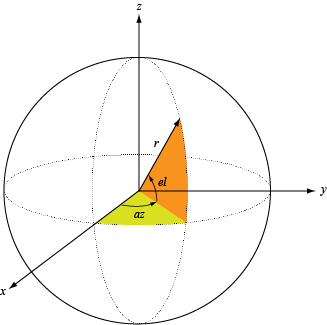# rcstruncone

Radar cross section of truncated cone

## Syntax

``rcspat = rcstruncone(r1,r2,height,c,fc)``
``rcspat = rcstruncone(r1,r2,height,c,fc,az,el)``
``[rcspat,azout,elout] = rcstruncone(___)``

## Description

example

````rcspat = rcstruncone(r1,r2,height,c,fc)` returns the radar cross section pattern of a truncated cone. `r1` is the radius of the small end of the cone, `r2` is the radius of the large end, and `height` is the cone height. The radar cross section is a function of signal frequency, `fc`, and signal propagation speed, `c`. You can create a non-truncated cone by setting `r1` to zero. The cone points downward towards the xy-plane. The origin is located at the apex of a the non-truncated cone constructed by extending the truncated cone to an apex.```

example

````rcspat = rcstruncone(r1,r2,height,c,fc,az,el)` also specifies the azimuth angles, `az`, and elevation angles, `el`, at which to compute the radar cross section.```

example

````[rcspat,azout,elout] = rcstruncone(___)` also returns the azimuth angles, `azout`, and elevation angles, `elout`, at which the radar cross sections are computed. You can use these output arguments with any of the previous syntaxes.```

## Examples

collapse all

Display the radar cross section (RCS) pattern of a truncated cone as a function of azimuth angle and elevation. The truncated cone has a bottom radius of 9.0 cm and a top radius of 12.5 cm. The cone height is 1 m. The operating frequency is 4.5 GHz.

Define the truncated cone geometry and signal parameters.

```c = physconst('Lightspeed'); fc = 4.5e9; radbot = 0.090; radtop = 0.125; hgt = 1;```

Compute the RCS for all directions using the default direction values.

```[rcspat,azresp,elresp] = rcstruncone(radbot,radtop,hgt,c,fc); imagesc(azresp,elresp,pow2db(rcspat)) xlabel('Azimuth Angle (deg)') ylabel('Elevation Angle (deg)') title('Truncated Cone RCS (dBsm)') colorbar```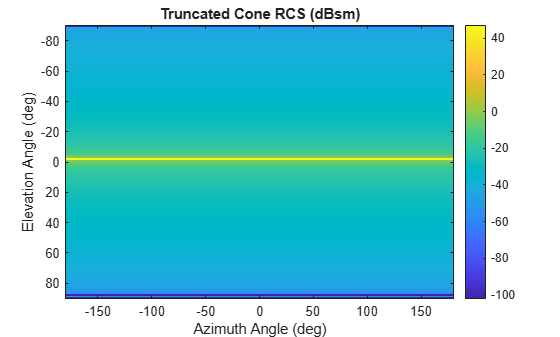Plot the radar cross section (RCS) pattern of a truncated cone as a function of elevation for a fixed azimuth angle of 5 degrees. The cone has a bottom radius of 9.0 cm and a top radius of 12.5 cm. The truncated cone height is 1 m. The operating frequency is 4.5.

Define the truncated cone geometry and signal parameters.

```c = physconst('Lightspeed'); fc = 4.5e9; radbot = 0.090; radtop = 0.125; hgt = 1;```

Compute the RCS at an azimuth angle of 5 degrees.

```az = 5.0; el = -90:90; [rcspat,azresp,elresp] = rcstruncone(radbot,radtop,hgt,c,fc,az,el); plot(elresp,pow2db(rcspat)) xlabel('Elevation Angle (deg)') ylabel('RCS (dBsm)') title('Truncated Cone RCS as Function of Elevation') grid on```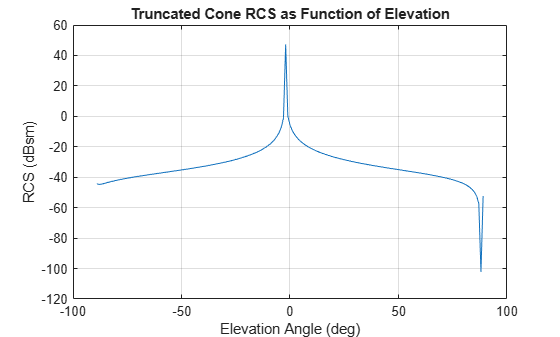Plot the radar cross section (RCS) pattern of a truncated cone as a function of frequency for a single direction. The cone has a bottom radius of 9.0 cm and a top radius of 12.5 cm. The truncated cone height is 1 m.

Specify the truncated cone geometry and signal parameters.

```c = physconst('Lightspeed'); radbot = 0.090; radtop = 0.125; hgt = 1;```

Compute the RCS over a range of frequencies for a single direction.

```az = 5.0; el = 20.0; fc = (100:100:4000)*1e6; [rcspat,azpat,elpat] = rcstruncone(radbot,radtop,hgt,c,fc,az,el); disp([azpat,elpat])```
``` 5 20 ```
```plot(fc/1e6,pow2db(squeeze(rcspat))) xlabel('Frequency (MHz)') ylabel('RCS (dBsm)') title('Truncated Cone RCS as Function of Frequency') grid on```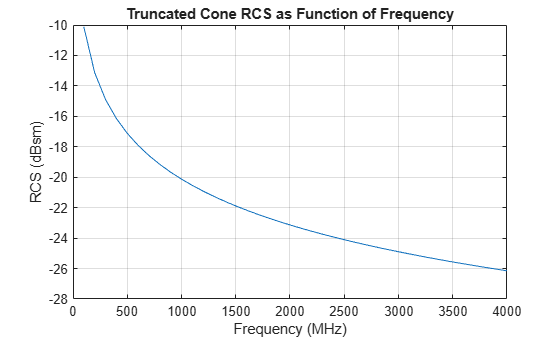Plot the radar cross section (RCS) pattern of a full cone as a function of elevation for a fixed azimuth angle. To define a full cone set the bottom radius to zero. Set the top radius to 20.0 cm and the cone height to 50 cm. Assume the operating frequency is 4.5 GHz and the azimuth angle is 5 degrees.

Define the cone geometry and signal parameters.

```c = physconst('Lightspeed'); fc = 4.5e9; radsmall = 0.0; radlarge = 0.20; hgt = 0.5;```

Compute the RCS for a fixed azimuth angle of 5 degrees.

```az = 5.0; el = -89:0.1:89; [rcspat,azresp,elresp] = rcstruncone(radsmall,radlarge,hgt,c,fc,az,el); plot(elresp,pow2db(rcspat)) xlabel('Elevation Angle (deg)') ylabel('RCS (dBsm)') title('Full Cone RCS as Function of Elevation') grid on```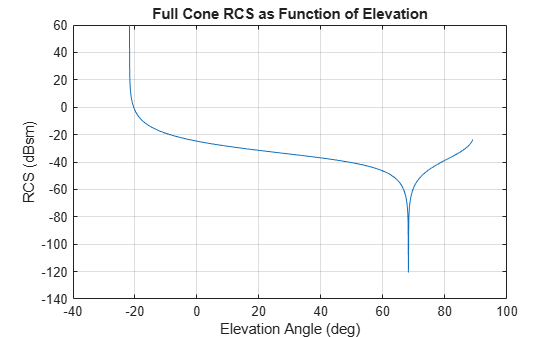## Input Arguments

collapse all

Radius of small end of truncated cone, specified as a nonnegative scalar. Units are in meters.

Example: `5.5`

Data Types: `double`

Radius of large end of truncated cone, specified as a positive scalar. Units are in meters.

Example: `5.5`

Data Types: `double`

Height of truncated cone, specified as a positive scalar. Units are in meters.

Example: `3.0`

Data Types: `double`

Signal propagation speed, specified as a positive scalar. Units are in meters per second. For the SI value of the speed of light, use `physconst('LightSpeed')`.

Example: `3e8`

Data Types: `double`

Frequency for computing radar cross section, specified as a positive scalar or positive, real-valued, 1-by-L row vector. Frequency units are in Hz.

Example: `[100e6 200e6]`

Data Types: `double`

Azimuth angles for computing directivity and pattern, specified as a real-valued 1-by-M row vector where M is the number of azimuth angles. Angle units are in degrees. Azimuth angles must lie between –180° and 180°, inclusive.

The azimuth angle is the angle between the x-axis and the projection of a direction vector onto the xy-plane. The azimuth angle is positive when measured from the x-axis toward the y-axis.

Example: `-45:2:45`

Data Types: `double`

Elevation angles for computing directivity and pattern, specified as a real-valued, 1-by-N row vector where N is the number of desired elevation directions. Angle units are in degrees. Elevation angles must lie between –90° and 90°, inclusive.

The elevation angle is the angle between a direction vector and xy-plane. The elevation angle is positive when measured towards the z-axis.

Example: `-75:1:70`

Data Types: `double`

## Output Arguments

collapse all

Radar cross section pattern, returned as a real-valued N-by-M-by-L array. N is the length of the vector returned in the `elout` argument. M is the length of the vector returned in the `azout` argument. L is the length of the `fc` vector. Units are in meters-squared.

Data Types: `double`

Azimuth angles for computing directivity and pattern, returned as a real-valued 1-by-M row vector where M is the number of azimuth angles specified by the `az` input argument. Angle units are in degrees.

The azimuth angle is the angle between the x-axis and the projection of the direction vector onto the xy-plane. The azimuth angle is positive when measured from the x-axis toward the y-axis.

Data Types: `double`

Elevation angles for computing directivity and pattern, returned as a real-valued 1-by-N row vector where N is the number of elevation angles specified in `el` output argument. Angle units are in degrees.

The elevation angle is the angle between the direction vector and xy-plane. The elevation angle is positive when measured towards the z-axis.

Data Types: `double`